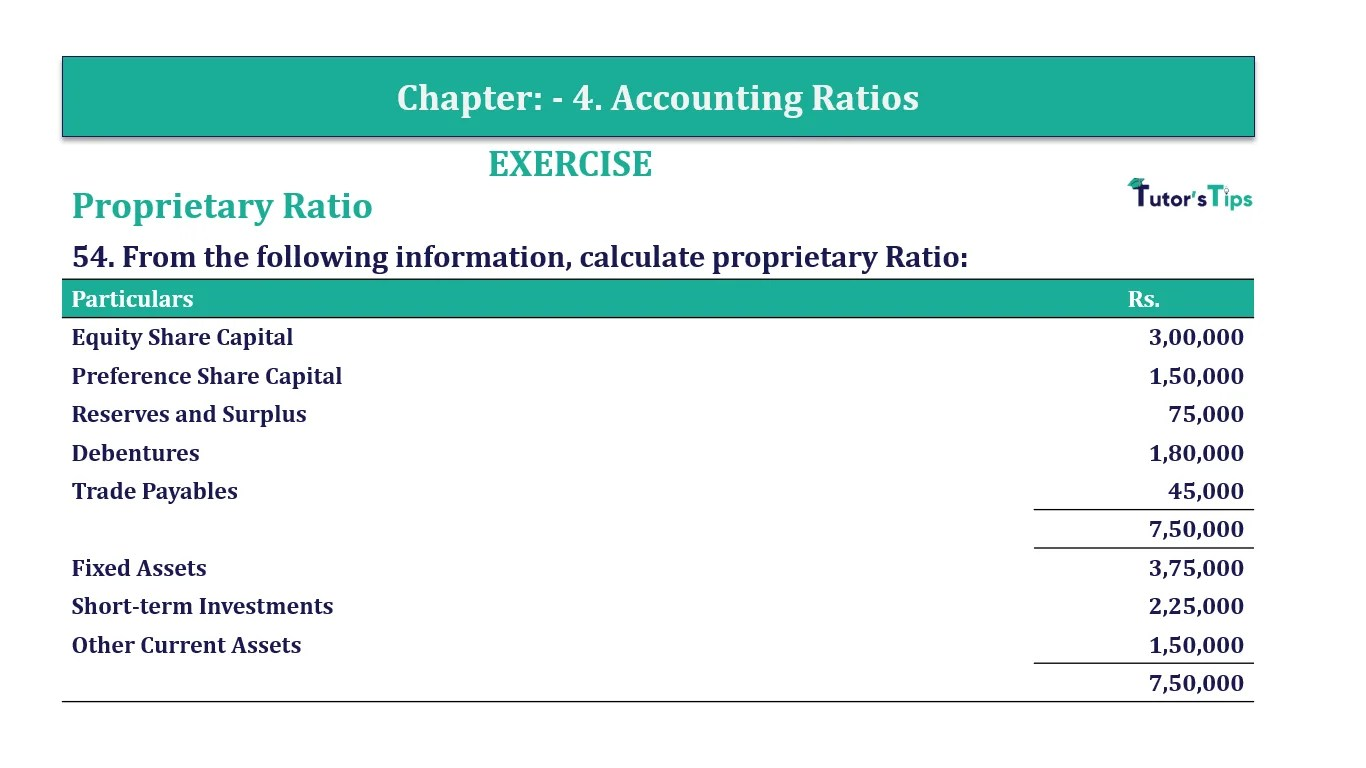# Question 54 Chapter 4 of +2-B – T.S. Grewal 12 ClassQuestion 54 Chapter 4 of +2-B

Proprietary Ratio

54. From the following information, calculate proprietary Ratio:

 Particulars Rs. Equity Share Capital 3,00,000 Preference Share Capital 1,50,000 Reserves and Surplus 75,000 Debentures 1,80,000 Trade Payables 45,000 7,50,000 Fixed Assets 3,75,000 Short-term Investments 2,25,000 Other Current Assets 1,50,000 7,50,000

### The solution of Question 54 Chapter 4 of +2-B: –

 Shareholders’ Funds = Equity Share Capital + Preference Share Capital + Reserves and Surplus = Rs.3,00,000 + Rs.1,50,000 + Rs.75,000 Shareholders’ Funds = Rs. 5,25,000 Total Assets = Fixed Assets + Current Assets + Short-term Investments = Rs.3,75,000 + Rs.1,50,000 + Rs.2,25,000 Total Assets = Rs. 7,50,000

 Total Assets to Debt Ratio = Total Assets = Rs.5,25,000 Total Assets Rs.7,50,000 = 0.7: 1

 Total Assets to Debt Ratio = Total Assets X 100 Total Assets
 Total Assets to Debt Ratio = Rs. 5,25,000 X 100 Rs. 7,50,000 = 70%

Balance Sheet: Meaning, Format & Examples

Comment if you have any question.

Also, Check out the solved question of previous Chapters: –